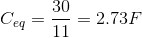# High School Physics : Calculating Total Capacitance

## Example Questions

### Example Question #3 : Capacitors

Three capacitors are in series. They have capacitances of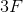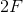, and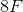, respectively. What is their total capacitance?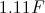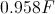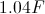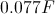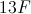Explanation:

For capacitors in series the formula for total capacitance is: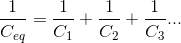Note that this formula is similar to the formula for total resistance in parallel. Using the values for each individual capacitor, we can solve for the total capacitance.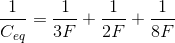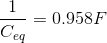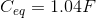### Example Question #1 : Calculating Total Capacitance

Three capacitors are in parallel. They have capacitance values of, and. What is their total capacitance?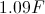Explanation:

For capacitors in parallel the formula for total capacitance is: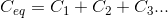Note that this formula is similar to the formula for total resistance in series. Using the values for each individual capacitor, we can solve for the total capacitance.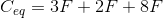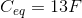### Example Question #5 : Capacitors

Three capacitors, each with a capacity of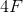are arranged in parallel. What is the total capacitance of this circuit?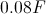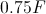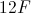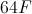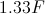Explanation:

The formula for capacitors in parallel is:Our three capacitors all have equal capacitance values. We can simply add them together to find the total capacitance.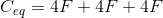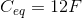### Example Question #6 : Capacitors

What is the total capacitance of a series circuit with capacitors of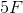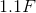, and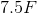?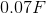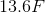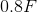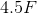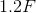Explanation:

The total capacitance of a series circuit isPlug in our given values.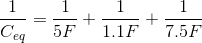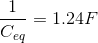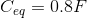### Example Question #7 : Capacitors

Calculate the total capacitance of a circuit with the following three capacitors in parallel.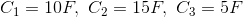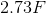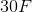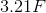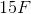Explanation:

To calculate the total capacitance for capacitors in parallel, simply sum the value of each individual capacitor.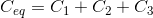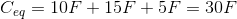### Example Question #8 : Capacitors

Calculate the total capacitance of a circuit with the following three capacitors in series.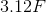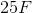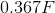Explanation:

To find the total capacitance for capacitors in series, we must sum the inverse of each individual capacitance and take the reciprocal of the result.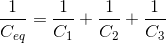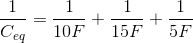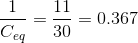Remember, you must still take the final reciprocal!